# Modelplotr v1.0 now on CRAN: Visualize the Business Value of your Predictive Models

2. Cumulative lift plot

The cumulative lift plot, often referred to as lift plot or index plot, helps you answer the question:

When we apply the model and select the best X ntiles, how many times better is that than using no model at all?

The lift plot helps you in explaining how much better selecting based on your model is compared to taking random selections instead. Especially when models are not yet used that often within your organisation or domain, this really helps business understand what selecting based on models can do for them.

The lift plot only has one reference line: the ‘random model’. With a random model we mean that each observation gets a random number and all cases are divided into ntiles based on these random numbers. When we would do that, the % of actual target category observations in each ntile would be equal to the overall % of actual target category observations in the total set. Since the lift is calculated as the ratio of these two numbers, we get a horizontal line at the value of 1. Your model should however be able to do better, resulting in a high ratio for ntile 1. How high the lift can get, depends on the quality of your model, but also on the % of target class observations in the data: If 50% of your data belongs to the target class of interest, a perfect model would 'only' do twice as good (lift: 2) as a random selection. With a smaller target class value, say 10%, the model can potentially be 10 times better (lift: 10) than a random selection. Therefore, no general guideline of a 'good' lift can be specified. Towards the last ntile, since the plot is cumulative, with 100% of cases, we have the whole set again and therefore the cumulative lift will always end up at a value of 1. It looks like this:To generate the cumulative lift plot for our gradient boosted trees model predicting term deposit buying, we call the function plot_cumlift(). Let's add some highlighting to see how much better a selection based on our model containing the best 20 (perce)ntiles would be, compared to a random selection of 20% of all customers:

```# plot the cumulative lift plot and annotate the plot at percentile = 20
plot_cumlift(data = plot_input,highlight_ntile = 20)
##
## Plot annotation for plot: Cumulative lift
## - When we select 20% with the highest probability according to model gradient boosted trees in test data, this selection for term.deposit cases is 4.3 times better than selecting without a model.
##
##
```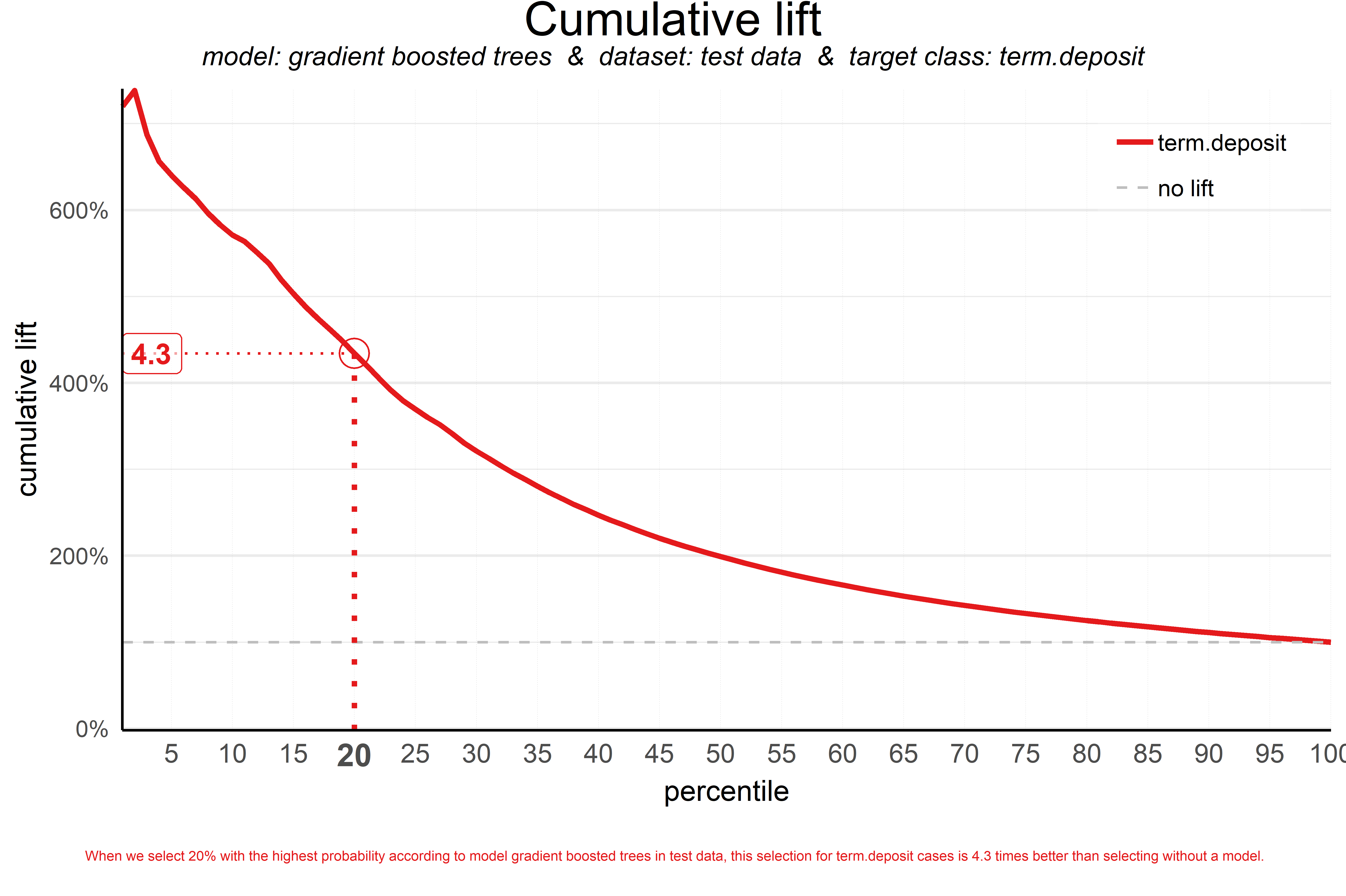A term deposit campaign targeted at a selection of 20% of all customers based on our gradient boosted trees model can be expected to have a 4 times higher response (434%) compared to a random sample of customers. Not bad, right? The cumulative lift really helps in getting a positive return on marketing investments. It should be noted, though, that since the cumulative lift plot is relative, it doesn't tell us how high the actual response will be on our campaign...

3. Response plot

One of the easiest to explain evaluation plots is the response plot. It simply plots the percentage of target class observations per ntile. It can be used to answer the following business question:

When we apply the model and select ntile X, what is the expected % of target class observations in that ntile?

The plot has one reference line: The % of target class cases in the total set. It looks like this: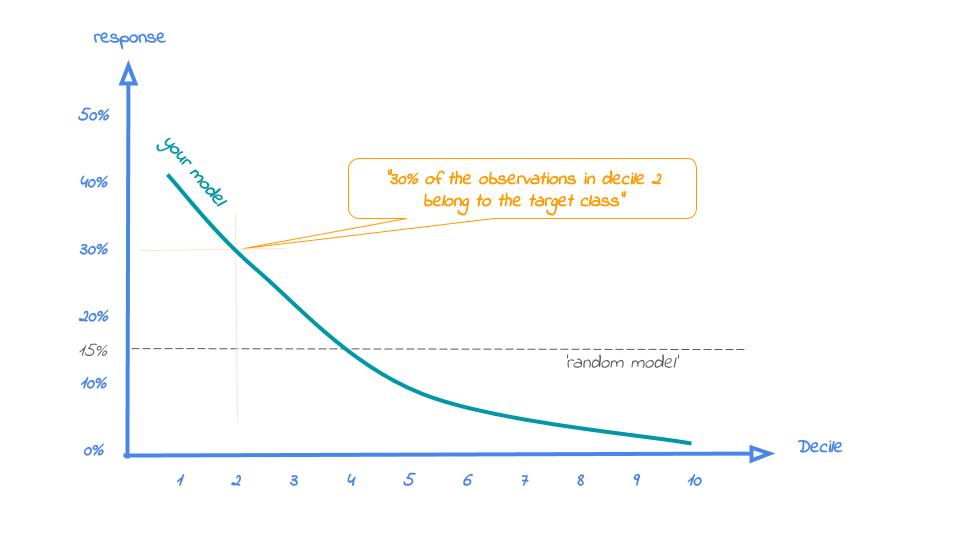A good model starts with a high response value in the first ntile(s) and suddenly drops quickly towards 0 for later ntiles. This indicates good differentiation between target class members - getting high model scores - and all other cases. An interesting point in the plot is the location where your model’s line intersects the random model line. From that ntile onwards, the % of target class cases is lower than a random selection of cases would hold.

To generate the response plot for our term deposit model, we can simply call the function plot_response(). Let's immediately highlight the plot to have the interpretation of the response plot at (perce)ntile 10 added to the plot:

```# plot the response plot and annotate the plot at ntile = 10
plot_response(data = plot_input,highlight_ntile = 10)
##
## Plot annotation for plot: Response
## - When we select ntile 10 according to model gradient boosted trees in dataset test data the % of term.deposit cases in the selection is 50.4%.
##
##
```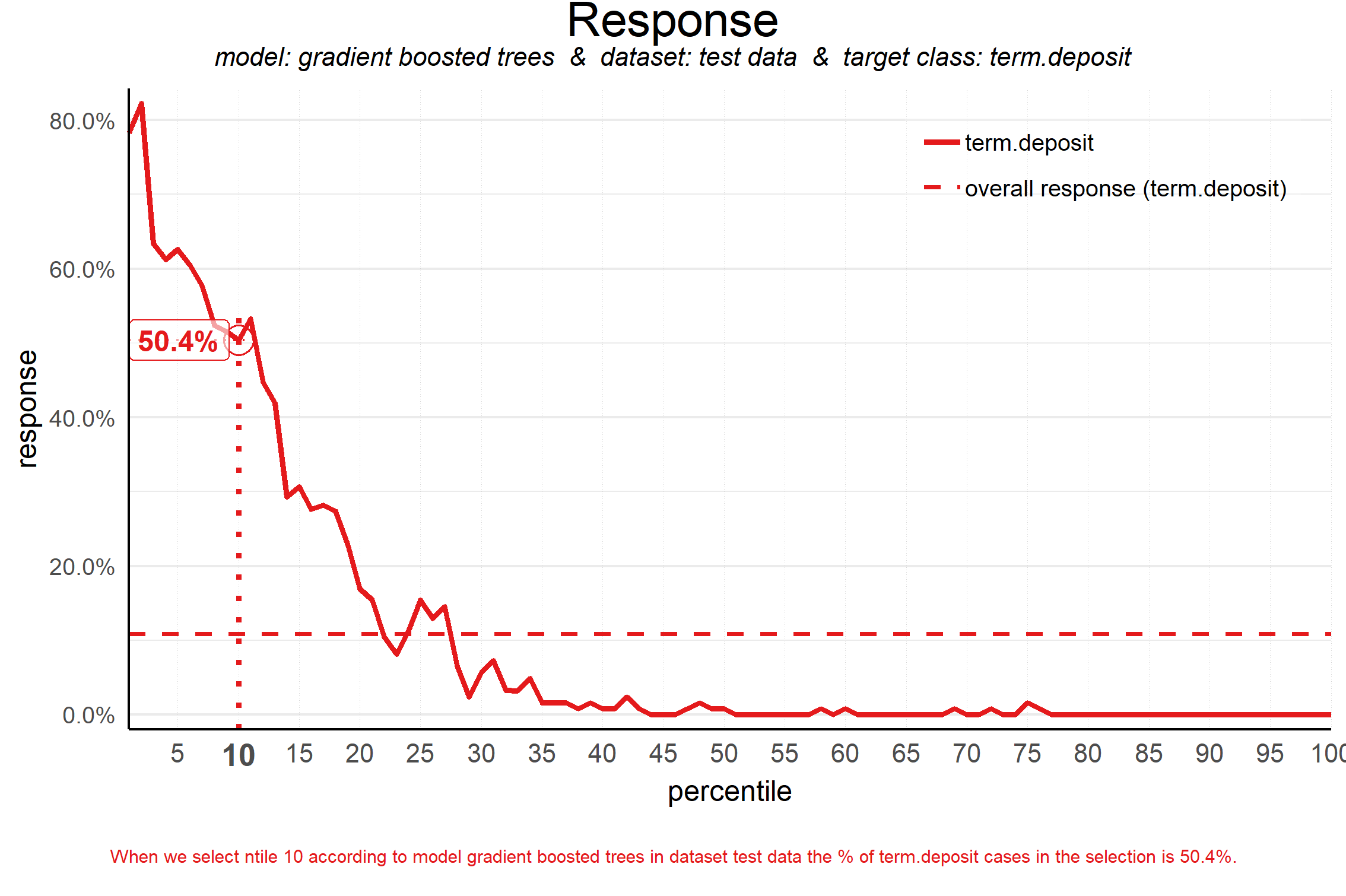As the plot shows and the text below the plot states: When we select decile 1 according to model gradient boosted trees in dataset test data the % of term deposit cases in the selection is 51%.. This is quite good, especially when compared to the overall likelihood of 12%. The response in the 20th ntile is much lower, about 10%. From ntile 22 onwards, the expected response is lower than the overall likelihood of 12%. However, most of the time, our model will be used to select the highest decile up until some decile. That makes it even more relevant to have a look at the cumulative version of the response plot. And guess what, that's our final plot!

4. Cumulative response plot

Finally, one of the most used plots: The cumulative response plot. It answers the question burning on each business reps lips:

When we apply the model and select up until ntile X, what is the expected % of target class observations in the selection?

The reference line in this plot is the same as in the response plot: the % of target class cases in the total set.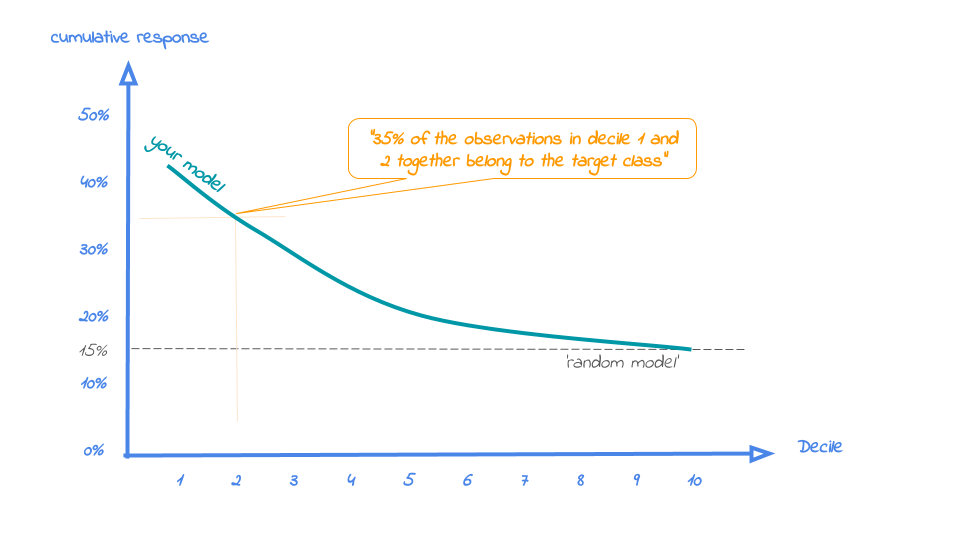Whereas the response plot crosses the reference line, in the cumulative response plot it never crosses it but ends up at the same point for the last ntile: Selecting all cases up until ntile 100 is the same as selecting all cases, hence the % of target class cases will be exactly the same. This plot is most often used to decide - together with business colleagues - up until what decile to select for a campaign.

Back to our banking business case. To generate the cumulative response plot, we call the function plot_cumresponse(). Let's highlight it at percentile 30 to see what the overall expected response will be if we select prospects for a term deposit offer based on our gradient boosted trees model:

```# plot the cumulative response plot and annotate the plot at decile = 3
plot_cumresponse(data = plot_input,highlight_ntile = 30)
##
## Plot annotation for plot: Cumulative response
## - When we select ntiles 1 until 30 according to model gradient boosted trees in dataset test data the % of term.deposit cases in the selection is 34.9%.
##
##
```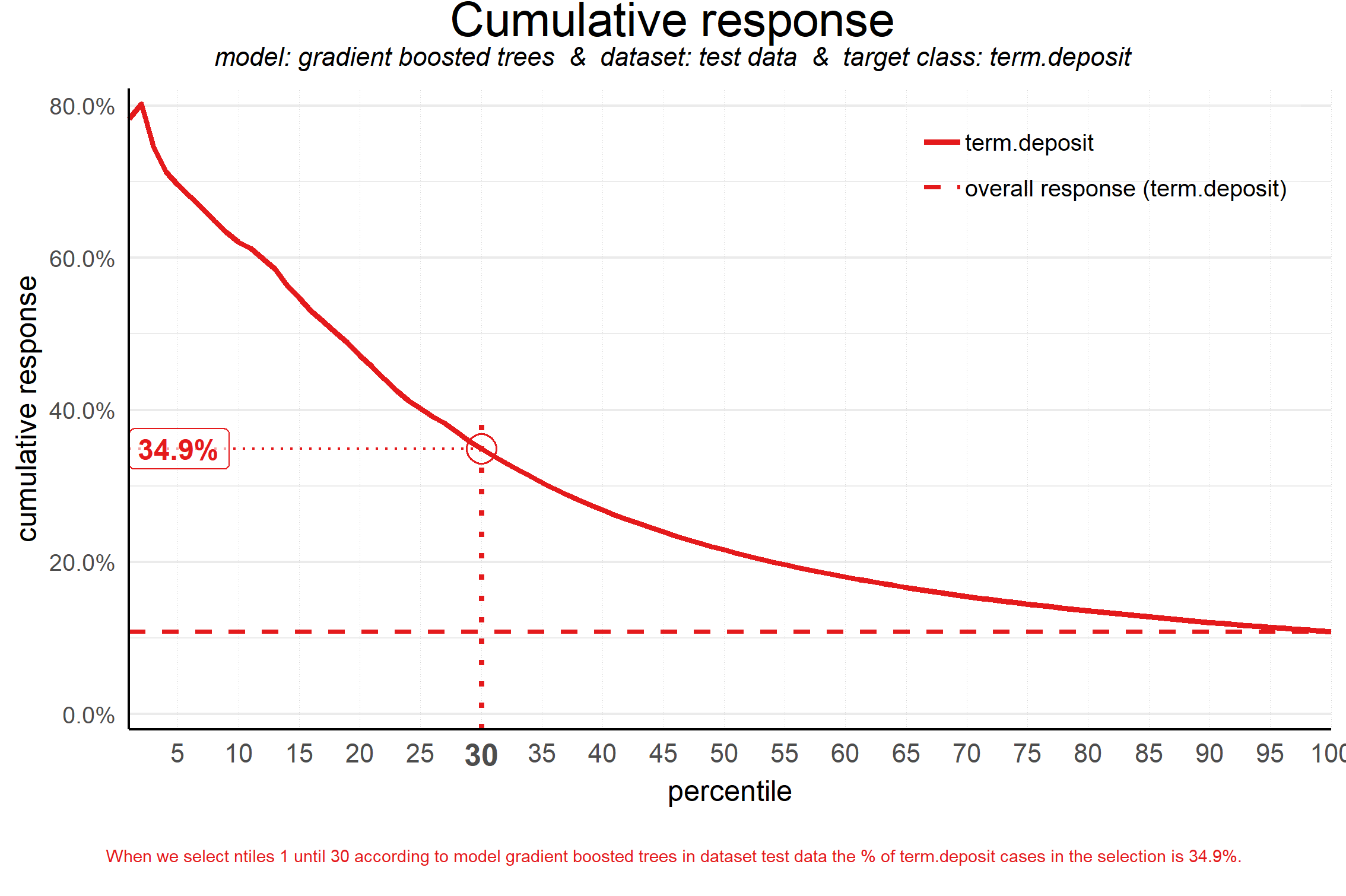When we select ntiles 1 until 30 according to model gradient boosted trees in dataset test data the % of term deposit cases in the selection is 36%. Since the test data is an independent set, not used to train the model, we can expect the response on the term deposit campaign to be 36%.

The cumulative response percentage at a given decile is a number your business colleagues can really work with: Is that response big enough to have a successful campaign, given costs and other expectations? Will the absolute number of sold term deposits meet the targets? Or do we lose too much of all potential term deposit buyers by only selecting the top 30%? To answer that question, we can go back to the cumulative gains plot. And that's why there's no absolute winner among these plots and we advice to use them all. To make that happen, there's also a function to easily combine all four plots.

All four plots together

With the function call plot_multiplot we get the previous four plots on one grid. We can easily save it to a file to include it in a presentation or share it with colleagues.

```# plot all four evaluation plots and save to file, highlight decile 2
plot_multiplot(data = plot_input,highlight_ntile=2,
save_fig = TRUE,save_fig_filename = 'Selection model Term Deposits')
##
## Plot annotation for plot: Cumulative gains
## - When we select 20% with the highest probability according to model gradient boosted trees, this selection holds 87% of all term.deposit cases in test data.
##
##
##
## Plot annotation for plot: Cumulative lift
## - When we select 20% with the highest probability according to model gradient boosted trees in test data, this selection for term.deposit cases is 4.3 times better than selecting without a model.
##
##
##
## Plot annotation for plot: Response
## - When we select ntile 2 according to model gradient boosted trees in dataset test data the % of term.deposit cases in the selection is 32.2%.
##
##
##
## Plot annotation for plot: Cumulative response
## - When we select ntiles 1 until 2 according to model gradient boosted trees in dataset test data the % of term.deposit cases in the selection is 47.2%.
##
##
## Warning: No location for saved plot specified! Plot is saved to tempdir(). Specify 'save_fig_filename' to customize location and name.
## Plot is saved as: C:\Users\J9AF3~1.NAG\AppData\Local\Temp\Rtmpc5waFv/Selection model Term Deposits.png
```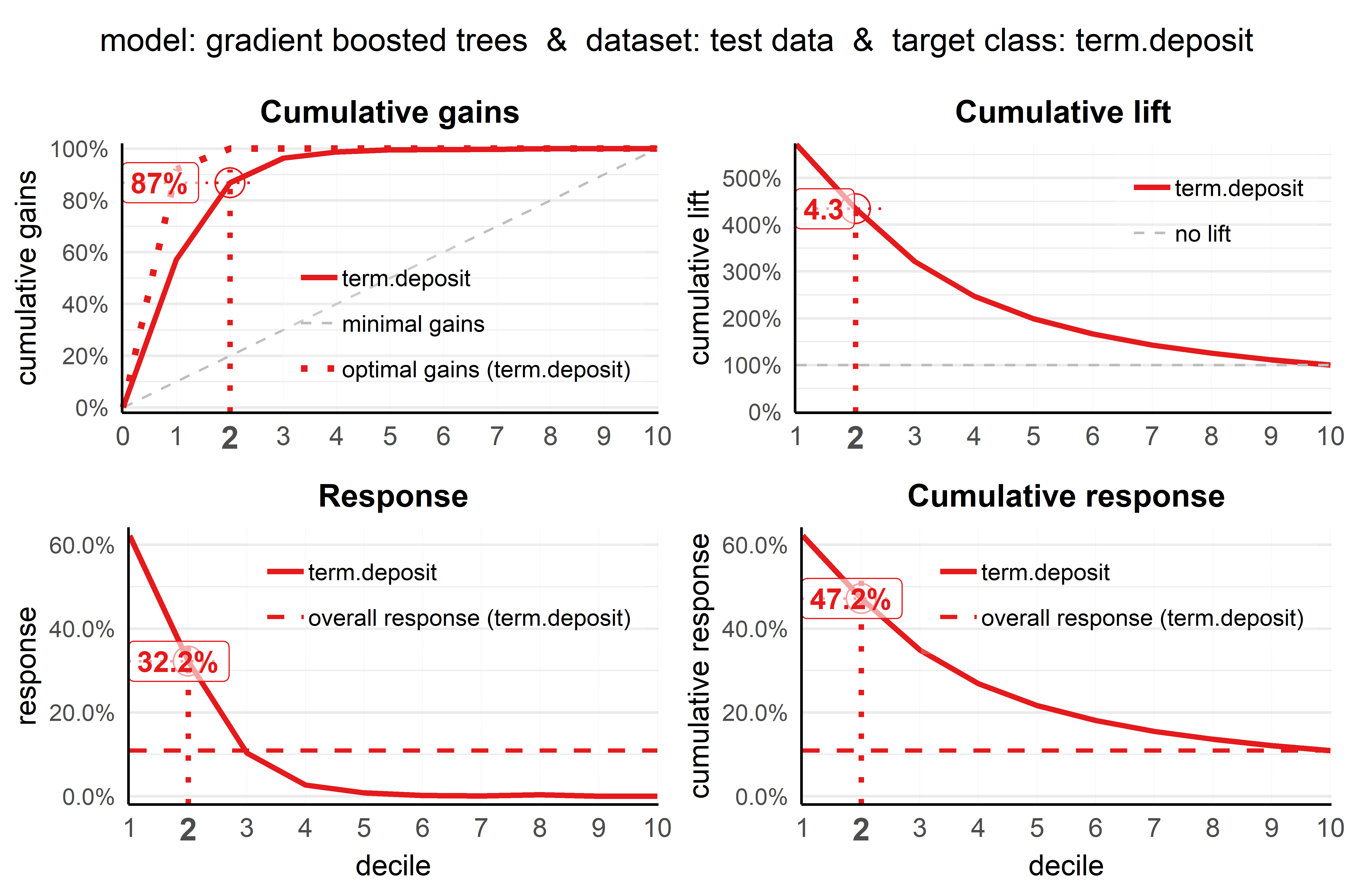And there's more! To plot the financial implications of implementing a predictive model, modelplotr provides three additional plots: the Costs & revenues plot, the Profit plot and the ROI plot. So, when you know what the fixed costs, variable costs and revenues per sale associated with a campaign based on your model are, you can use these to visualize financial consequences of using your model. Here, we'll just show the Profit plot. See the package vignette for more details on the Costs & Revenues plotand the Return on Investment plot.

The profit plot visualized the cumulative profit up until that decile when the model is used for campaign selection. It can be used to answer the following business question:

When we apply the model and select up until ntile X, what is the expected profit of the campaign?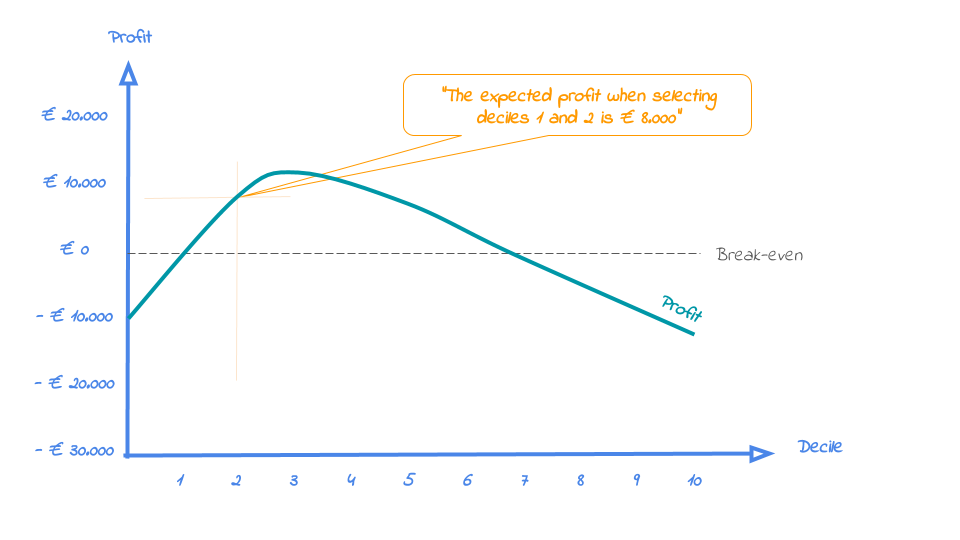From this plot, it can be quickly spotted with what selection size the campaign profit is maximized. However, this does not mean that this is the best option from an investment point of view.

Business colleagues should be able to tell you the expected costs and revenues regarding the campaign. Let's assume they told us fixed costs for the campaign (a tv commercial and some glossy print material) are in total € 75,000 and each targeted customer costs another € 50 (prospects are called and receive an incentive) and the expected revenue per new term deposit customer is € 250 according to the business case.

```#Profit plot - automatically highlighting the ntile where profit is maximized!
plot_profit(data=plot_input,fixed_costs=75000,variable_costs_per_unit=50,profit_per_unit=250)
##
## Plot annotation for plot: Profit
## - When we select ntiles 1 until 19 in dataset test data using model gradient boosted trees to target term.deposit cases the expected profit is <U+20AC>93,850
##
##
```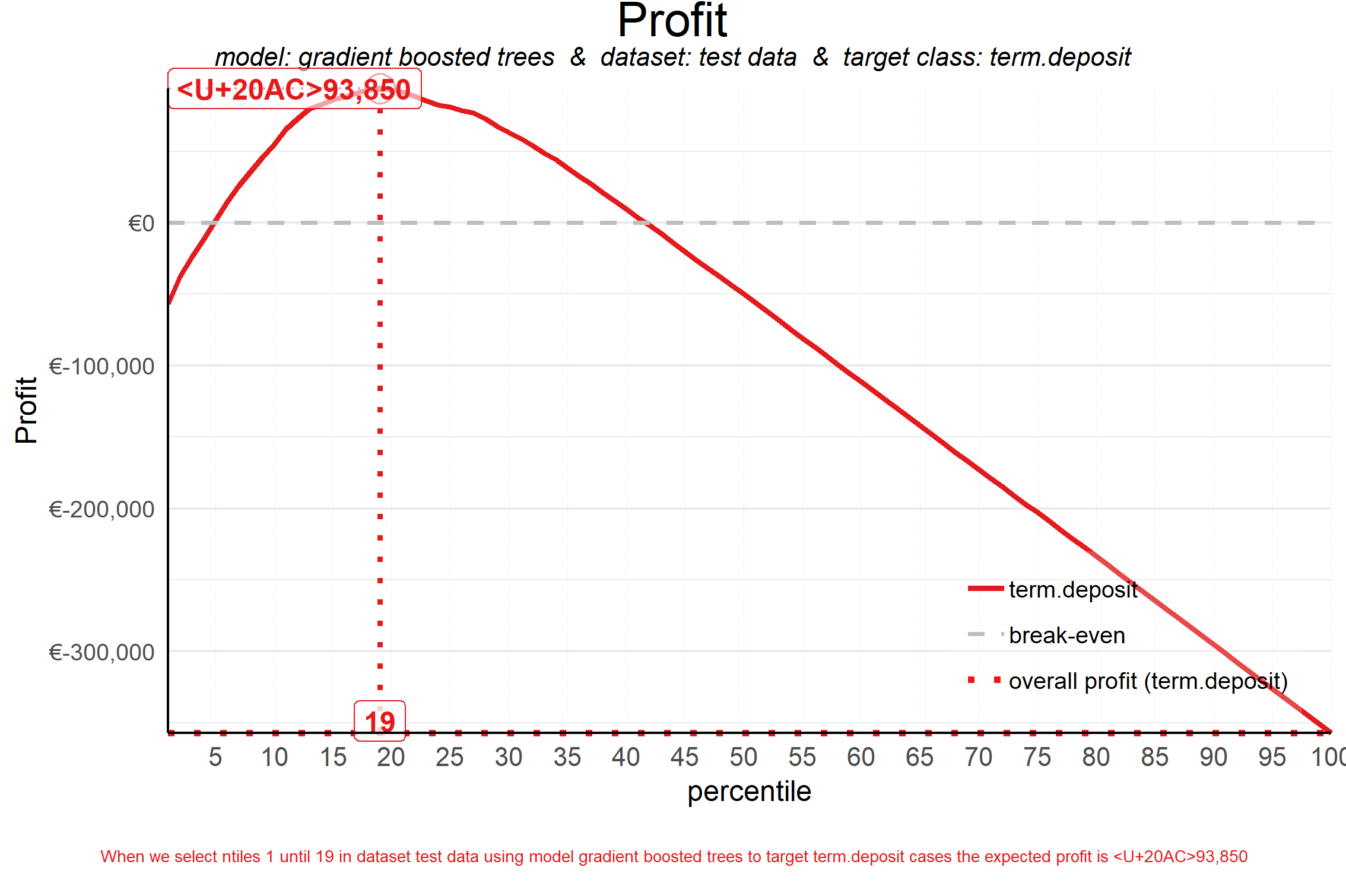Using this plot, we can decide to select the top 19 ntiles according to our model to maximize profit and earn about € 94,000 with this campaign. Both decreasing and increasing the selection based on the model would harm profits, as the plot clearly shows.

Neat! With these plots, we are able to talk with business colleagues about the actual value of our predictive model, without having to bore them with technicalities any nitty gritty details. We've translated our model in business terms and visualised it to simplify interpretation and communication. Hopefully, this helps many of you in discussing how to optimally take advantage of your predictive model building efforts.

### Get more out of modelplotr: using different scopes, highlight ntiles, customize text & colors.

As we mentioned earlier, the modelplotr package also enables to make interesting comparisons, using the scope parameter. Comparisons between different models, between different datasets and (in case of a multiclass target) between different target classes. Also, modelplotr provides a lot of customization options: You can highlight plots and add annotation texts to explain the plots, change all textual elements in the plots and customize line colors. Curious? Please have a look at the package documentation or read our other posts on modelplotr.

However, to give one example, we could compare whether gradient boosted trees was indeed the best choice to select the top-30% customers for a term deposit offer:

```# set plotting scope to model comparison
plot_input <- plotting_scope(prepared_input = scores_and_ntiles,scope = "compare_models")
## Data preparation step 2 succeeded! Dataframe created.
## "prepared_input" aggregated...
## Data preparation step 3 succeeded! Dataframe created.
##
## Models "random forest", "multinomial logit", "gradient boosted trees", "artificial neural network" compared for dataset "test data" and target value "term.deposit".

# plot the cumulative response plot and annotate the plot at ntile 20
plot_cumresponse(data = plot_input,highlight_ntile = 20)
##
## Plot annotation for plot: Cumulative response
## - When we select ntiles 1 until 20 according to model random forest in dataset test data the % of term.deposit cases in the selection is 46.5%.
## - When we select ntiles 1 until 20 according to model multinomial logit in dataset test data the % of term.deposit cases in the selection is 42.1%.
## - When we select ntiles 1 until 20 according to model gradient boosted trees in dataset test data the % of term.deposit cases in the selection is 47.2%.
## - When we select ntiles 1 until 20 according to model artificial neural network in dataset test data the % of term.deposit cases in the selection is 40.7%.
##
##
```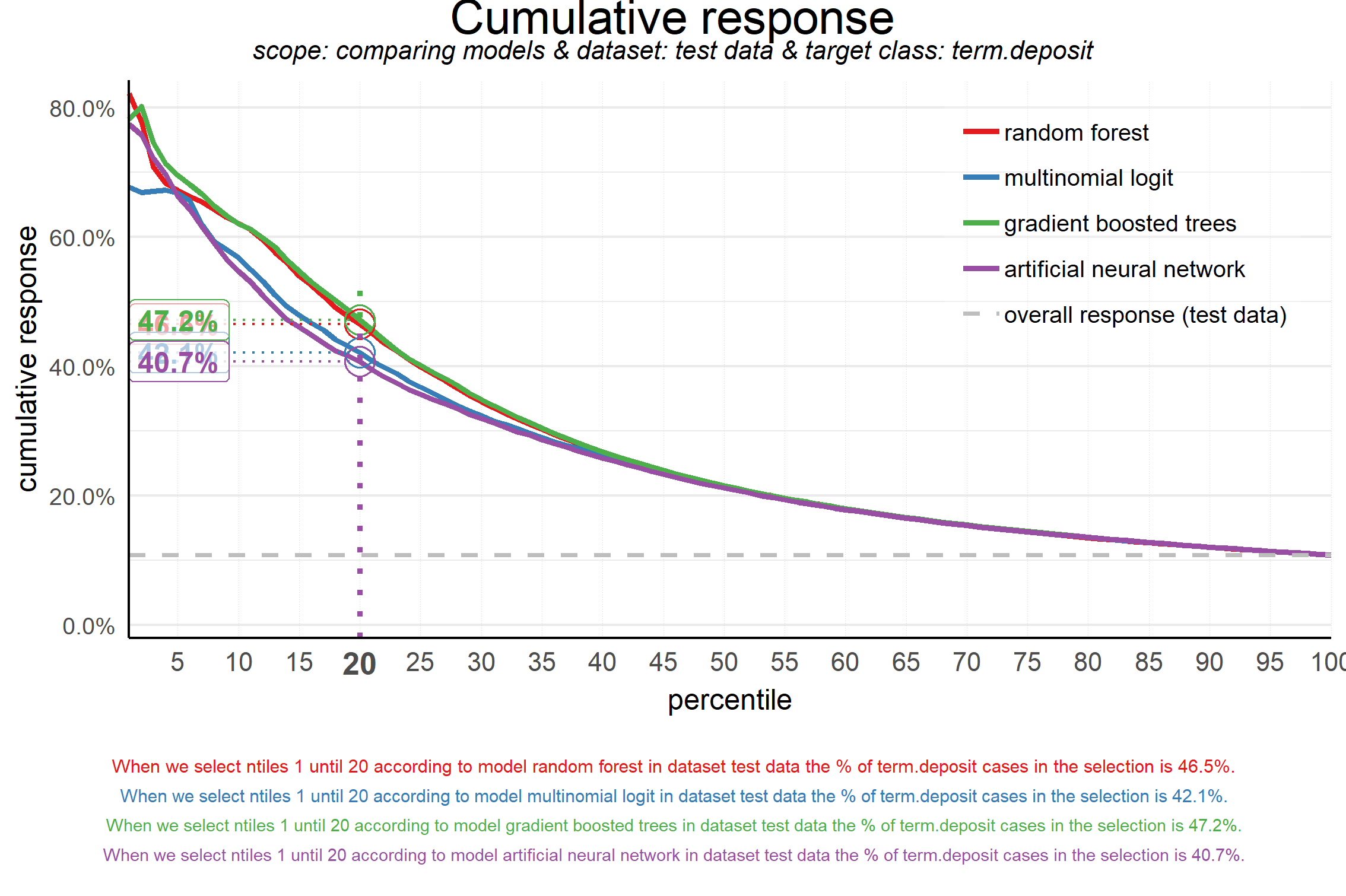Seems like the algorithm used will not make a big difference in this case. Hopefully you agree by now that using these plots really can make a difference in explaining the business value of your predictive models!

You can read more on how to use modelplotr here. In case you experience issues when using modelplotr, please let us know via the issues section on Github. Any other feedback or suggestions, please let us know via jurriaan.nagelkerke@gmail.com or pb.marcus@hotmail.com. Happy modelplotting!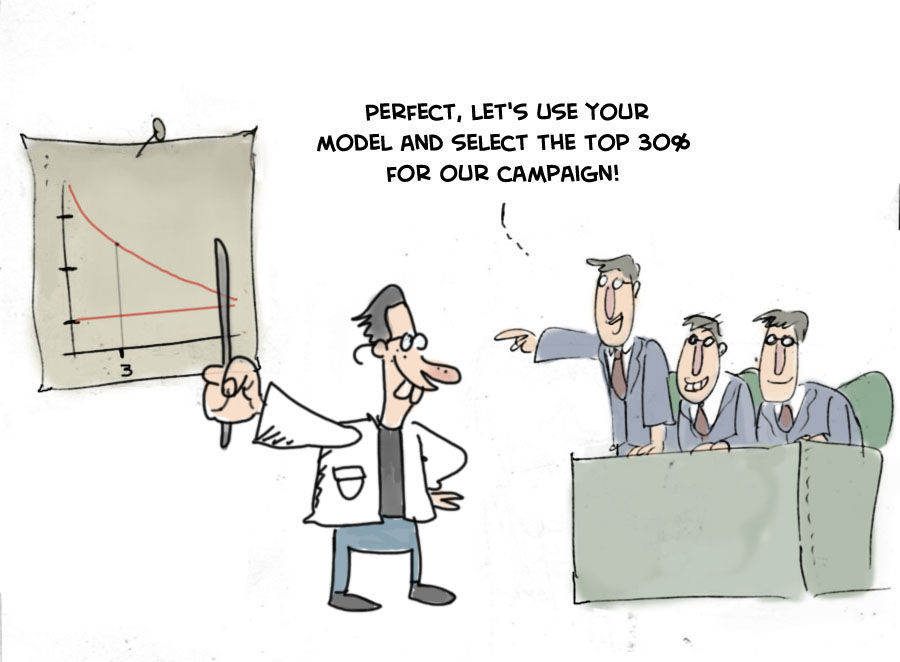Jurriaan Nagelkerke is a Consultant in Advanced Analytics & Data Science @ Cmotions (Dutch consultancy firm focused on advanced analytics @ Data science).

Pieter Marcus is a Data Scientist @ De Persgroep Nederland (biggest publisher in the Netherlands)

Original. Reposted with permission.

Related: